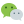# 二、按位与（&）

`1 & 1 = 1` , `0 & 1 = 0` , ` 0 & 0 = 0`

```   0 1 1   ---> 3
& 1 0 1   ---> 5
--------
0 0 1   ---> 1
```

# 三、按位或 （|）

`1 | 1 = 1` , `1 | 0 = 1` , `0 | 0 = 0`

```   0 1 1   ---> 3
| 1 0 1   ---> 5
--------
1 1 1   ---> 7
```

# 四、按位异或（^）

`1 ^ 1 = 0` , ` 1 ^ 0 = 1` , `0 ^ 0 = 0`

```   0 1 1    ---> 3
^ 1 0 1    ---> 5
---------
1 1 0    ---> 6
```

## 简单应用

### 1、交换变量值

`a ^ b ^a = b` , `a ^ b ^ b =a`

``````#include <stdio.h>
int main()
{
int a = 3, b = 5;
int temp; /*定义一个临时变量用于交换方便*/
temp = a;
a = b;
b = temp;
printf("a = %d, b = %d", a, b); /*输出结果为：a = 5, b = 3 实现了变量值的交换*/
}
``````

``````#include <stdio.h>
int main()
{
int a = 3, b = 5;
a ^= b; /*等同于：a = a ^ b 之后会讲*/
b = a ^ b; /*这时 a ^ b 等于 原来 a 的值*/
a ^= b; /*a = a ^ b*/
printf("a = %d, b = %d", a, b);
}
``````

a, b 为负数时同样适用，但位运算只适用于整型，浮点数不可用

### 2、简单加密

``````5201314 ^ 1998
``````

``````5200492 ^ 1998 这样就生成了原数字：`5201314`.
``````

# 五、按位取反（~）

`~ 1 = 0` , `~ 0 = 1`

``` ~ 00000000 00000000 00000000 00000101   ---> 5
---------------------------------------
11111111 11111111 11111111 11111010   ---> -6
```

# 六、移位运算

## 1、左移位（«）

`需要移位的数据 << 需要移动的位数`

```  00000000 00000000 00000000 00000101   ---> 5
-------------------------------------
00000000 00000000 00000000 00010100   ---> 20
```

## 2、右移位

`需要移位的数据 >> 需要移动的位数`

```  00000000 00000000 00000000 00000101   ---> 5
-------------------------------------
00000000 00000000 00000000 00000001   --->  1
```

```  11111111 11111111 11111111 11111010    ---> -6
-------------------------------------
11111111 11111111 11111111 11111110    ---> -2
```

# 七、复合赋值符

`a &= b` 等价于 `a = a & b`

# 返回顶部

### 评论：微信公众号：程序骑士